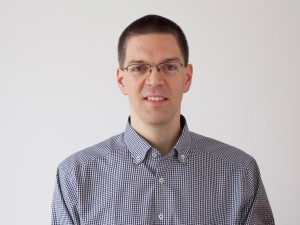## Solving Hard Quantum Problems: Everything is ConnectedQuantum systems are extremely hard to analyse if they consist of more than just a few parts. It is not difficult to calculate a single hydrogen atom, but in order to describe an atom cloud of several thousand atoms, it is usually necessary to use rough approximations. The reason for this is that quantum particles are connected to each other and cannot be described separately. Kaspar Sakmann (TU Wien, Vienna) and Mark Kasevich (Stanford, USA) have now shown in an article published in “Nature Physics” that this problem can be overcome. They succeeded in calculating effects in ultra-cold atom clouds which can only be explained in terms of the quantum correlations between many atoms. Such atom clouds are known as Bose-Einstein condensates and are an active field of research.

Quantum Correlations
Quantum physics is a game of luck and randomness. Initially, the atoms in a cold atom cloud do not have a predetermined position. Much like a die whirling through the air, where the number is yet to be determined, the atoms are located at all possible positions at the same time. Only when they are measured, their positions are fixed. “We shine light on the atom cloud, which is then absorbed by the atoms”, says Kaspar Sakmann. “The atoms are photographed, and this is what determines their position. The result is completely random.”There is, however, an important difference between quantum randomness and a game of dice:  if different dice are thrown at the same time, they can be seen as independent from each other. Whether or not we roll a six with die number one does not influence the result of die number seven. The atoms in the atom cloud on the other hand are quantum physically connected. It does not make sense to analyse them individually, they are one big quantum object. Therefore, the result of every position measurement of any atom depends on the positions of all the other atoms in a mathematically complicated way.

“It is not hard to determine the probability that a particle will be found at a specific position”, says Kaspar Sakmann. “The probability is highest in the centre of the cloud and gradually diminishes towards the outer fringes.” In a classically random system, this would be all the information that is needed. If we know that in a dice roll, any number has the probability of one sixth, then we can also determine the probability of rolling three ones with three dice. Even if we roll five ones consecutively, the probability remains the same the next time. With quantum particles, it is more complicated than that.

“We solve this problem step by step”, says Sakmann. “First we calculate the probability of the first particle being measured on a certain position. The probability distribution of the second particle depends on where the first particle has been found. The position of the third particle depends on the first two, and so on.” In order to be able to describe the position of the very last particle, all the other positions have to be known. This kind of quantum entanglement makes the problem mathematically extremely challenging.

Only Correlations Can Explain the Experimental Data
But these correlations between many particles are extremely important – for example for calculating the behaviour of colliding Bose-Einstein-condensates. “The experiment shows that such collisions can lead to a special kind of quantum waves. On certain positions we find many particles, on an adjacent position we do not find any”, says Kaspar Sakmann. “If we consider the atoms separately, this cannot be explained. Only if we take the full quantum distribution into account, with all its higher correlations, these waves can be reproduced by our calculations.”

Also other phenomena have been calculated with the same method, for instance Bose-Einstein-condensates which are stirred with a laser beam, so that little vortices emerge – another typical quantum many-particle-effect. “Our results show how important theses correlations are and that it is possible to include them in quantum calculations, in spite of all mathematical difficulties”, says Sakmann. With certain modifications, the approach can be expected to be useful for many other quantum systems as well.